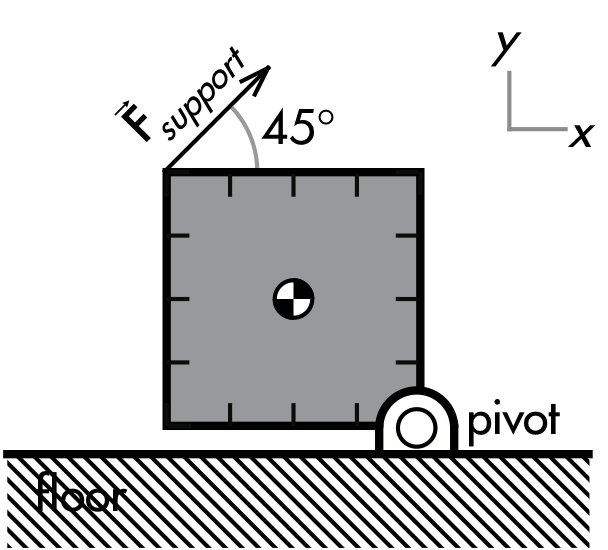## 20191104

### Physics quiz question: comparing torques on supported square

Physics 205A Quiz 5, fall semester 2019
Cuesta College, San Luis Obispo, CAA uniform 0.40 m × 0.40 m square with  a mass of 0.50 kg is pivoted at one corner. It is supported by a 45° diagonal force at the opposite corner such that the bottom edge is parallel to the ground. Calculate all torques with respect to the corner pivot. The torque exerted by the __________ has a greater magnitude. (A) weight force.
(B) support force.
(C) (There is a tie.)
(D) (Not enough information is given.)

Correct answer (highlight to unhide): (C)

Since the square is in static equilibrium ("is supported") and does not rotate, then the net torque on it is equal to zero (Στ = 0), such that the counterclockwise torque of the weight force on the square must equal the clockwise torque of the support force on the square:

(ccw) τw = (cw) τFsupport.

Sections 70854, 70855
Exam code: quiz05Gu1L
(A) : 23 students
(B) : 21 students
(C) : 7 students
(D) : 0 students

Success level: 13%
Discrimination index (Aubrecht & Aubrecht, 1983): 0.24